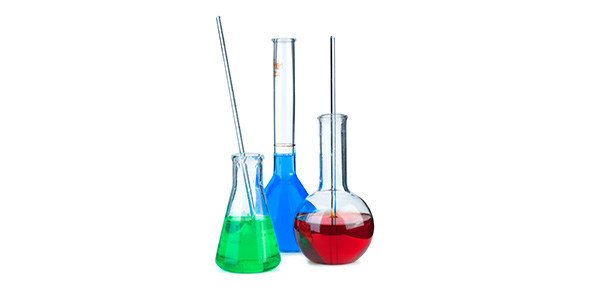10 Questions | Total Attempts: 151SettingsChemists deserve respect, as the content that they study is not a walk in the park. Among the many things that they are expected to master are chemical elements and compounds. The chemical quiz below tests on this and more.

Related Topics
• 1.
The volume of water that must be added in order to dilute 40 mL of 9.0 M HCl to a concentration of 6.0 M is closest to
• A.

10 mL

• B.

20 mL

• C.

30 mL

• D.

40 mL

• E.

60 mL

• 2.
A chemical supply company sells a concentrated solution of aqueous  (molar mass 98 g/mol) that is 50. percent by mass.  At 25C, the density of the solution is 1.4 g/mL.  What is the molarity of the  solution at 25C?
• A.

1.8 M

• B.

3.6 M

• C.

5.1 M

• D.

7.1 M

• 3.
A 360. mg sample of aspirin, , (molar mass 180 g), is dissolved in enough water to produce 200. mL of solution.  What is the molarity of aspirin in a 50. mL sample of this solution?
• A.

0.0800 M

• B.

0.0400 M

• C.

0.0200 M

• D.

0.0100 M

• E.

0.00250 M

• 4.
Appromixately what mass of (250 g/mol) is required to prepare 250 mL of 0.10 M copper (II) sulfate solution?
• A.

4.0 g

• B.

6.2 g

• C.

34 g

• D.

85 g

• E.

140 g

• 5.
• A.

Pb to the power of plus 2 end exponent (aq)

• B.

Zn to the power of plus 2 end exponent (aq)

• C.

CrO subscript 4 to the power of minus 2 end exponent (aq)

• D.

SO subscript 4 to the power of minus 2 end exponent (aq)

• E.

OH to the power of minus 1 end exponent (aq)

• 6.
Oxygen is acting as an oxidizing agent in all of the following reactions EXCEPT
• A.

2 C (s) + O2 (g) --> 2 CO (g)

• B.

S (s) + O2 (g) --> SO2 (g)

• C.

2 F2 (g) + O2 (g) --> 2 OF2 (g)

• D.

2 Na (s) + O2 (g) --> Na2O2 (s)

• E.

2 Mg (s) + O2 (g) --> 2 MgO (s)

• 7.
If 200. mL of 0.60 M (aq) is added to 400. mL of distilled water, what is the concentration of (aq) in the resulting solution?  (Assume volumes are additive.)
• A.

0.20 M

• B.

0.30 M

• C.

0.40 M

• D.

0.60 M

• E.

1.20 M

• 8.
What mass of Au is produced with 0.0500 mol of is reduced completely with excess ?
• A.

9.85 g

• B.

19.7 g

• C.

24.5 g

• D.

39.4 g

• E.

48.9 g

• 9.
Which of the following is a weak acid in aqueous solution?
• A.

HCl

• B.

HClO subscript 4

• C.

HNO subscript 3

• D.

H subscript 2 S

• E.

H subscript 2 SO subscript 4

• 10.
• A.

SnF subscript 2

• B.

SnSO subscript 4

• C.

Sn(SO subscript 4 ) subscript 2

• D.

ZnF

• E.

ZnF subscript 2